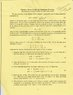# EC Air resistance problem.

• errubio
In summary, the student is struggling with understanding the problem and does not know where the e comes from. They are also familiar with vectors and how to add them together and with splitting a vector into horizontal and vertical components. They are attempting to solve the problem but are not sure how to get to the solution. They think about the problem and realize that the e comes from the limit as n approaches infinity of (1+r/n)^1/n. They relate this to euler's method and realize that this is a solution to the problem.

## Homework Statement

all information is on picture

## The Attempt at a Solution

I read the paper and understood it but when I got to the first question I was really confused. I do not even know what the problem is asking. This problem could give me an A in the class but its confusing the hell out of me. where did the e come from. If anyone can give me any hints or anything I would really appreciate it. I have worked on it for about 2 hours and I cannot figure it out. Please help

#### Attachments

•Scan.jpg
33.7 KB · Views: 401
I end up with Vx=e^(-Yt) where Y=b\m
But i don't know where the cosine comes from for part 1

errubio said:
I end up with Vx=e^(-Yt) where Y=b\m
But i don't know where the cosine comes from for part 1

Are you familiar with adding vectors together and with splitting a vector into horizontal and vertical components?

for the first part, where

V,x(t)=V0costheta(e^-gamma(t)) I would search up the true definition of e. I believe it has to do with taking the limit as n->infinity of (1 +r/n)^1/n, but I think I'm wrong. Once you gather this information, think about it's meaning and relate it to this problem to prove that this is true.

oops I was close:
e = limn->infinity (1 + 1/n)^n.
http://mathforum.org/dr.math/faq/faq.e.html
where let's say you have V0 then V=V0(1+a/n)^nt if P=P0(1+r/n)^nt=P0(e^rt)due to the limit as n approaches infinity.

Don't quote me on this one, but just try to reason this analysis out to provide a thoughtful proof that makes logical sense.

I'd then relate this analysis to euler's method. You know what that is right? I mean I hardly do, but this limit as n approaches infinity seems analogous to euler's method.